# Perform Trajectory Tracking and Compute Joint Torque for Manipulator Using Simscape

This example shows you how to use Simulink® with Robotics System Toolbox™ to perform trajectory tracking and compute joint torque required to drive the Simscape™ Multibody™ model of the manipulator along the given joint trajectory. This example can be further extended to scenarios with obstacles to observe the changes in torque profile.

Robotics System Toolbox, Simscape Multibody, and Robotics System Toolbox Robot Library Data support package are required to run this example.

### Introduction

In this example, you will load an included robot model using `loadrobot` as a `rigidBodyTree` object, then create a Simscape Multibody model of the robot using `smimport`. Configure the model to accept motion inputs such as joint position, velocity, and acceleration generated using the Trapezoidal Velocity Profile Trajectory block and return the computed joint torque. Simulate the model to visualize the robot motion and plot the joint torques.

### Load Robot Model in Workspace

This example uses a model of the KINOVA® Gen3, a 7 degree-of-freedom robot manipulator. Call `loadrobot` to generate a `rigidBodyTree` model of the robot. Set the `DataFormat` properties to be consistent with Simscape.

`robot = loadrobot("kinovaGen3",DataFormat="column");`

### Generate Simscape Multibody Model from Rigid Body Tree

Import the `robot` object into Simscape Multibody and get the model parameters.

```robotSM = smimport(robot,ModelName="ManipulatorTrajectoryPlanning_Subsystem"); sm_mdl = get_param(robotSM,"Name");```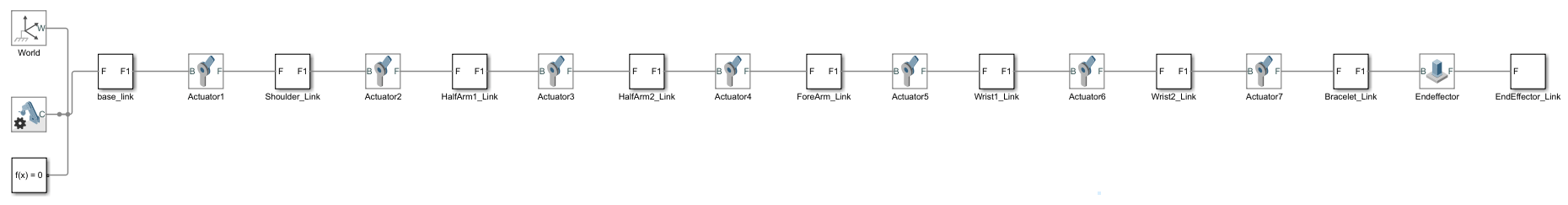### Configure Simscape Multibody Model

Prepare the Simscape Multibody model to accept the joint motion inputs and return the joint torques. You can follow the steps below to manually configure the model or use the `helperInstrumentSMModels` helper function to automatically configure the model.

#### Manual Configuration of Simscape Multibody Model

1. In your model, double-click a Joint block. The Property Inspector dialog box opens.

2. In the Property Inspector dialog box, select Z Revolute Primitive (Rz) > Actuation > Torque > Automatically computed, and select Z Revolute Primitive (Rz) > Actuation > Motion > Provided by Input. The block exposes a physical signal input port, labeled `q`.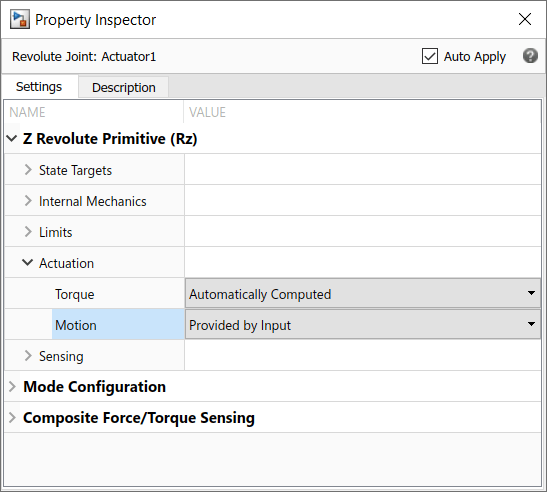3. In the Property Inspector block dialog box, select Z Revolute Primitive (Rz) > Sensing > Actuator Torque. The block exposes a physical signal output port, labeled `t`.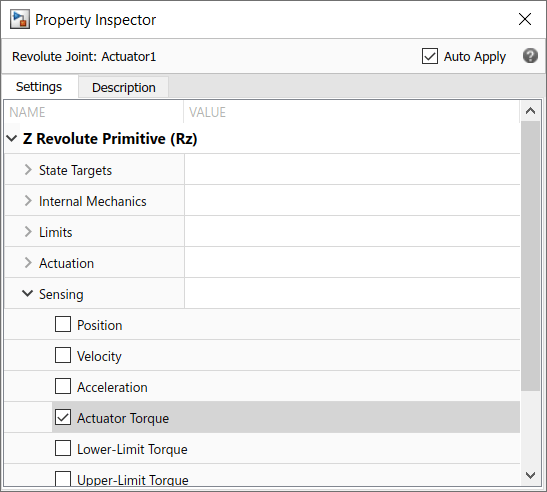4. Add a Simulink-PS Converter block from the Simscape > Utilities library, connect the Simulink-PS Converter block to physical signal input port `q` of the Joint block.

5. Double-click the Simulink-PS Converter block to open the block dialog box, click Input Handling Tab, select Provided signals > Input and first two derivatives.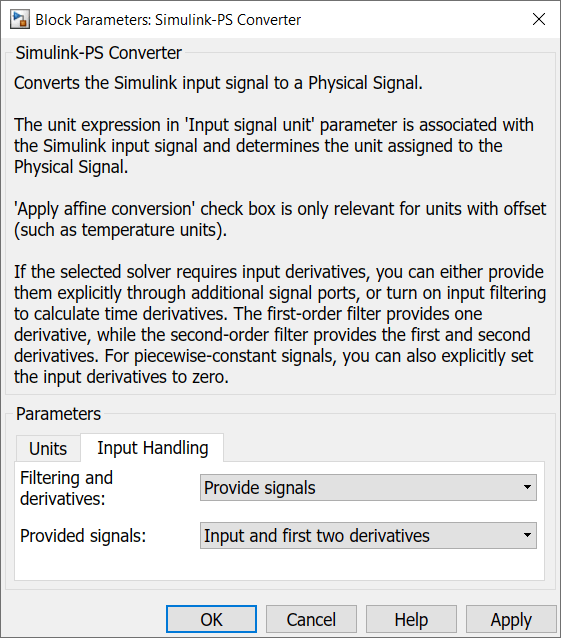6. Add three From block from the Simulink > Signal Routing library to the three input ports of the Simulink-PS Converter block.

7. Add PS-Simulink Converter block from the Simscape > Utilities library, connect the PS-Simulink Converter block to physical signal output port `t` of the Joint block.

8. Add Goto block from the Simulink > Signal Routing library to the output port of the PS-Simulink Converter block.9. Repeat these steps for all the Joint blocks.

10. Add three Demux blocks from the Simulink > Signal Routing library and connect the joint motions Goto blocks related to the respective joint motions From blocks.

11. Add a Mux block from the Simulink > Signal Routing library and connect the joint torque From blocks related to the respective joint torque Goto blocks.

12. Create a subsystem of the Simscape Multibody model.

#### Configure Simscape Multibody Model Using Helper Function

Use the `helperInstrumentSMModels` helper function to automatically configure the model.

Call the helper function to automatically configure the Simscape Multibody model to accept motion inputs such as joint position, velocity, and acceleration.

`helperInstrumentSMModels.instrumentRBTSupportedJointInputs(sm_mdl,robot,"motion")`

Call the helper function again to configure the Simscape Multibody model to attach torque sensors that measure torque at each joint.

`helperInstrumentSMModels.instrumentRBTSupportedJointOutputs(sm_mdl,robot,"torque")`

Create a subsystem of the Simscape Multibody model.

`helperInstrumentSMModels.convertToSubsystem(sm_mdl)`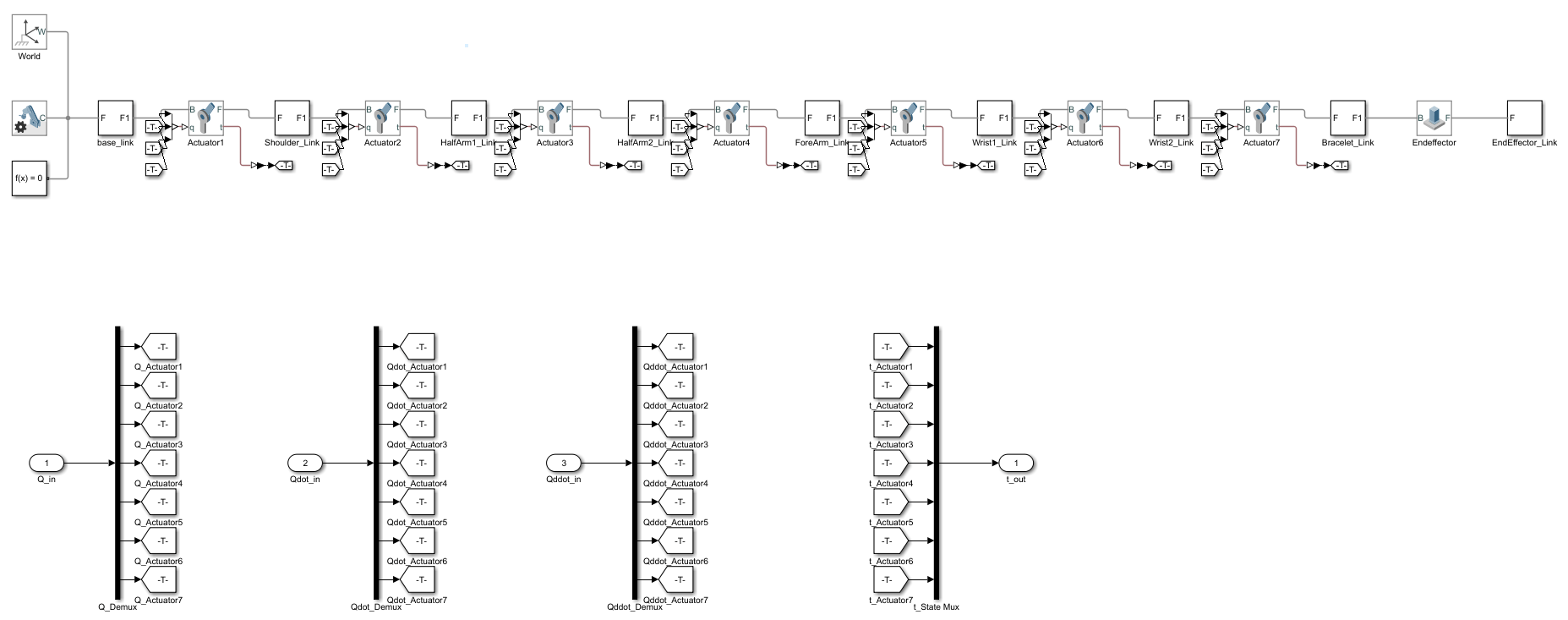#### Set Up Variables in Model Workspace

Set up the variables in the model workspace that specify the start and end waypoints, and the joint starting position and velocity.

```mdlWks = get_param(robotSM,'ModelWorkspace'); assignin(mdlWks,"robotToTest",robot) assignin(mdlWks,"q0",robot.homeConfiguration) assignin(mdlWks,"dq0",zeros(size(robot.homeConfiguration)))```

### Trajectory Generation

Add a Trapezoidal Velocity Profile Trajectory block from the Robotics System Toolbox > Utilities library to the model and connect the `q`, `qd`, and `qdd` ports of the block to the `Q_in`, `Qdot_in`, and `Qddot_in` input ports of the Simscape Multibody model subsystem.

In the Trapezoidal Velocity Profile Trajectory block parameters dialog box,

1. Set the Waypoints as `[robotToTest.homeConfiguration robotToTest.randomConfiguration robotToTest.randomConfiguration]`.

2. Set the Number of parameters to `1` and set the Parameter 1 as End Time.

3. Specify the End Time as `3`.### Final Setup

Add a Clock block from the Simulink > Sources library, connect the Clock block to the `Time` input port of the Trapezoidal Velocity Profile Trajectory block. Add a Scope block and connect the `t_out` port of the Simscape Multibody model subsystem to the Scope input.### Simulate Model

Open the provided ManipulatorTrajectoryPlanning.slx model and replace the Robot subsystem with the subsystem created in ManipulatorTrajectoryPlanning_Subsystem model above, for it to be able to fetch the meshes correctly.

```open_system("ManipulatorTrajectoryPlanning.slx") ```

Save the model and simulate it. The Trapezoidal Velocity Profile Trajectory block generates random trajectories and drives the manipulator model accordingly. The manipulator model computes the joint torque required to execute the motion.

```sim("ManipulatorTrajectoryPlanning.slx","StopTime","4") ```

Visualize the multibody model in the Mechanics Explorer.Visualize the joint torques in the Scope.